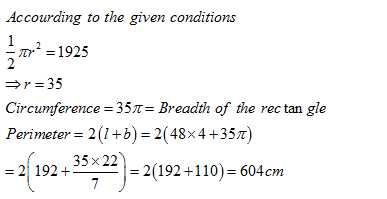• This is an assessment test.
• These tests focus on geometry and mensuration and are meant to indicate your preparation level for the subject.
• Kindly take the tests in this series with a pre-defined schedule.

## Geometry and Mensuration: Test 9

Congratulations - you have completed Geometry and Mensuration: Test 9. You scored %%SCORE%% out of %%TOTAL%%. You correct answer percentage: %%PERCENTAGE%% . Your performance has been rated as %%RATING%%
 Question 1
The circumference of two circles is 88 metres and 220 metres respectively. What is the difference between the area of the larger circle and the smaller circle?
 A 3422 sq. metre B 3242 sq. metre C 3424 sq. metre D None
Question 1 Explanation:
$\displaystyle \begin{array}{l}Let\text{ }the\text{ }two\text{ }circles\text{ }be\text{ }of\text{ }radii\text{ }r\text{ }and\text{ }R\text{ }cm\text{ }respectively.\\2\pi r=88\\r=14cm\\and\,\,2\pi R=220\\R=35cm\\Difference\text{ }in\text{ }area=\pi \left( {{R}^{2}}-{{r}^{2}} \right)=\frac{22}{7}\times 49\times 21=3234c{{m}^{2}}\end{array}$
 Question 2
What is the area of a circle whose radius is equal to the side of a square whose perimeter is 112 metres?
 A 176 sq. m. B 2504 sq. m. C 284 sq. m. D None
Question 2 Explanation:
$\displaystyle \begin{array}{l}Side\text{ }of\text{ }the\text{ }square~=\text{ }112/4\text{ }=\text{ }28\text{ }m.\\Area\,\,of\,\,circle\,\,\,=\pi \times {{28}^{2}}=\frac{22}{7}\times 28\times 28=2464{{m}^{2}}\\Correct\,\,option\,\,is\,\,e\end{array}$
 Question 3
The circumference of a semicircle of area 1925 sq. cm is equal to the breadth of a rectangle. If the length of the rectangle is equal to the perimeter of a square of side 48 cm, what is the perimeter of the rectangle?
 A 734 cm B 754 cm C 604 cm D 750 cm
Question 3 Explanation:Question 4
The total area of a circle and a rectangle is equal to 1166 sq.cm. The diameter of the circle is 28 cms. What is the sum of the circumference of the circle and the perimeter of the rectangle if the length of the rectangle is 25 cm?
 A 186 cm B 182 cm C 184 cm D Cannot be determined
Question 4 Explanation:
$\displaystyle \begin{array}{l}Area\text{ }of\text{ }the\text{ }circle=\pi \times {{14}^{2}}=196\times \frac{22}{7}=616c{{m}^{2}}\\Area\text{ }of\text{ }rectangle=1166-616=550c{{m}^{2}}\\Length\text{ }of\text{ }rectangle=25cm\\Thus\,breadth=\frac{550}{25}=22cm\\Sum\,\,of\,\,perimeter=2\pi r+2\left( 25+22 \right)\\=(2\pi \times 14)+94=182cm\end{array}$
 Question 5
The sum of the circumference of a circle and the perimeter of a square is equal to 272 cm. The diameter of the circle is 56 cm. What is the sum of the area of the circle and the area of the square?
 A 2464 sq. cm. B 2644 sq. cm. C 3040 sq. cm. D Cannot be determined
Question 5 Explanation:
$\begin{array}{l}2\pi r+4a=272\\\Rightarrow a=\frac{1}{4}\left( 272-176 \right)\\=\frac{1}{4}\times 96cm=24cm\\Sum\text{ }of\text{ }the\text{ }area=\pi {{r}^{2}}+{{a}^{2}}=2464+576=3040\end{array}$
Once you are finished, click the button below. Any items you have not completed will be marked incorrect.
There are 5 questions to complete.
 ← List →